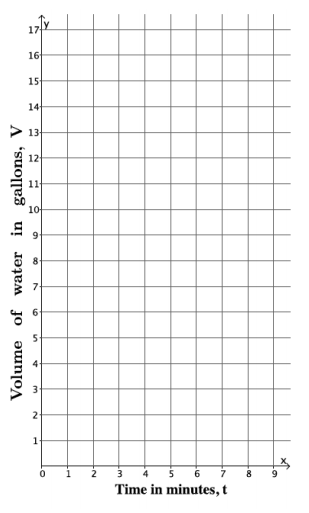# Linear Relationships

## Objective

Graph proportional relationships and interpret slope as the unit rate.

## Common Core Standards

### Core Standards

?

• 8.EE.B.5 — Graph proportional relationships, interpreting the unit rate as the slope of the graph. Compare two different proportional relationships represented in different ways. For example, compare a distance-time graph to a distance-time equation to determine which of two moving objects has greater speed.

?

• 6.EE.C.9

• 7.RP.A.2

## Criteria for Success

?

1. Draw graphs for proportional relationships in the coordinate plane.
2. Identify the slope of the graph and interpret it in context as the unit rate of the situation.

## Tips for Teachers

?

The following materials may be helpful for this lesson: graph paper.

#### Fishtank Plus

• Problem Set
• Student Handout Editor
• Vocabulary Package

## Anchor Problems

?

### Problem 1

Water flows at a constant rate out of a faucet. Suppose the volume of water that comes out in three minutes is 10.5 gallons.1. Write a linear equation to represent the volume of water, $v$, that comes out of the faucet in $t$ minutes.
2. Find the volume of water out of the faucet after 0 minutes, 1 minute, and 4 minutes.
3. Graph the equation in the coordinate plane.
4. What is the slope of the graph? What does it mean as a unit rate?

#### References

EngageNY Mathematics Grade 8 Mathematics > Module 4 > Topic B > Lesson 11Example 2

Grade 8 Mathematics > Module 4 > Topic B > Lesson 11 of the New York State Common Core Mathematics Curriculum from EngageNY and Great Minds. © 2015 Great Minds. Licensed by EngageNY of the New York State Education Department under the CC BY-NC-SA 3.0 US license. Accessed Dec. 2, 2016, 5:15 p.m..

Modified by The Match Foundation, Inc.

### Problem 2

Nia and Trey both had a sore throat so their mom told them to gargle with warm salt water.

• Nia mixed 1 teaspoon salt with 3 cups water.
• Trey mixed ${1\over 2}$ teaspoon salt with 1${{1\over2}}$cups of water.

Nia tasted Trey’s salt water. She said,
“I added more salt so I expected that mine would be more salty, but they taste the same.”

1. Explain why the salt water mixtures taste the same.
2. Find an equation that relates $s$, the number of teaspoons of salt, with $w$, the number of cups of water, for both of these mixtures.
3. Draw the graph of your equation from part (b).
4. Your graph in part (c) should be a line. Interpret the slope as a unit rate.

#### References

Illustrative Mathematics Sore Throats, Variation 2

Sore Throats, Variation 2, accessed on Feb. 23, 2018, 11:02 p.m., is licensed by Illustrative Mathematics under either the CC BY 4.0 or CC BY-NC-SA 4.0. For further information, contact Illustrative Mathematics.

## Problem Set

?The following resources include problems and activities aligned to the objective of the lesson that can be used to create your own problem set.

?

At the grocery store, sliced turkey deli meat is on sale for \$11 for 2 pounds.

1. What is the cost per pound of sliced turkey deli meat?
2. Write an equation to represent the cost, $y$, of sliced turkey deli meat measured in $x$ pounds.
3. Draw a graph of the situation.
4. What is the meaning of the slope of the line that you drew?

?